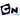## 寻觅CSDN Number

Tue, 22nd October 2019Edit on GithubbashCSDN Number

# 简介

medie2005在csdn论坛提出一个问题:

n=237 379 286 772 731 308 578 588 912 877 240 596 445 141 325 762 163 750 564 487 723 891 992 461 222 648 851 843 630 125 607 599 752 729 038 353 369 033 808 597 579 540 472 923 130 204 730 174 179 441 751 910 786 430 758 685 776 002 714 186 782 580 881 746 115 354 635 619

# 详细内容

gxqcn对上面苦涩难懂的内容做了进一步解读

$10^b + 1$ 分解因数，假设素数 $p_1 \le p_2 \le \dots \le p_r$ 均在其中， 如果将这$r$个素数依次排列成的十进制数恰好为一个b位素数 $P = \overline{p_1p_2\dots p_r}$ ， 那么$n = p_1\times p_2\times\dots\times p_r\times P$ 即为解之一。

gxqcn从网络上找到了$10^n\pm 1$因子分解的现成结果

	211
241
2161
2689
9091
459691
909091
4147571
29970369241
1661378260814161
265212793249617641
18276168846821336356291

J.K. Andersen再次出手，引用了同样的方法在2008年12月构造了更多的结果

medie2005另外非常期望能够找到$\frac{Factor(n)}n$尽量小的结果。到现在我们还只能找到$\frac{Factor(n)}n=11$的结果

n=859 × 1058313049 × 8591058313049
Factor(n)=85910583130498591058313049
=8591058313049 × (10^13+1)
Factor(n)/n=11 

Github